• 用DAC0832产生锯齿波电压 #include<reg51.h> //包含单片机寄存器的头文件 #include<absacc.h> //包含对片外存储器地址进行操作的头文件 sbit CS=P2^7; //将CS位定义为P2.7引脚 sbit WR12=P3^6; //将WR12位定义为P3.6...
• ## C语言，锯齿数组

千次阅读 2012-08-05 14:21:49
#include #include int main(void) {  int **a,b,c,i;  printf("请输入行数:");  scanf("%d",&b);//b是行数  a=(int **)malloc(sizeof(int **)*b);    if (a==NULL) {   fprintf(stderr,"分配...
#include <stdio.h> #include <stdlib.h>
int main(void) {  int **a,b,c,i;
printf("请输入行数:");
scanf("%d",&b);//b是行数
a=(int **)malloc(sizeof(int **)*b);    if (a==NULL) {   fprintf(stderr,"分配失败");
exit(1);
}    for (i = 0; i<b; i++) {   printf("请输入第%d行的元素个数:",i+1);    scanf("%d",&c);//C是输入的元素个数    a[i]=(int *)malloc(sizeof(int)*(c+1));/*为第i行分配c+1个int空间，多出来的一个位置用于保存这一行的长度*/    if (a[i]==NULL) {     fprintf(stderr,"分配失败");     exit(1);    }    a[i]=c;          /*将第i行的长度保存到第i行的第一个元素中*/    for (c=1; c<=a[i]; c++) {     a[i][c]=1;   }  }
for (i = 0; i<b; i++) {      for (c=1; c<=a[i]; c++) {        printf("%d",a[i][c]);   }
putchar('\n');     free(a[i]); /*释放为第i行分配的空间*/  }    free(a);  /*释放为a分配的空间*/    return 0; }
展开全文c 语言 include null
• C语言三角波、正弦波、整流正弦波、三角波、锯齿波发生器
• 输出4种波形：方波、锯齿波、三角波和正弦波；2.幅值调节范围为：2V-10V;3.频率调节范围为：20Hz-80Hz;内容简介信号发生器仿真系统由51单片机最小系统、LCD12864液晶模块、DAC0832波形转换模块（包括波形幅值与频率...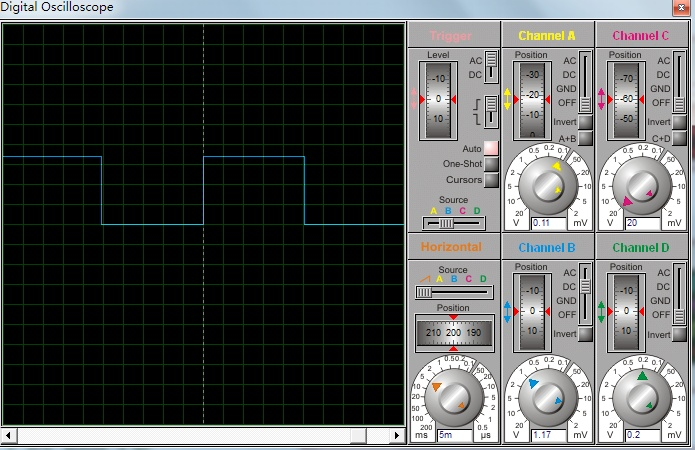设计要求1.输出4种波形：方波、锯齿波、三角波和正弦波；2.幅值调节范围为：2V-10V;3.频率调节范围为：20Hz-80Hz;
内容简介信号发生器仿真系统由51单片机最小系统、LCD12864液晶模块、DAC0832波形转换模块（包括波形幅值与频率转换）、按键控制模块以及示波器测量模块构成。通过Keil平台下的C语言编程，单片机控制输出的数字信号经工作在直通方式下的DAC0832数模转换，输出方波、正弦波、锯齿波、三角波4种电压波形，且波形幅值、频率可调。波形的切换、频率和幅值的增减可通过按键模块实现，同时当前的波形信息可实时更新在LCD12864显示模块上，波形形象可通过示波器测量模块展现。整个设计由Proteus仿真实现，并能满足设计内容及要求。
本设计内容详细，涵盖 单片机最小系统设计原理、按键与LCD12864液晶设计、DAC0832幅值控制电路、频率控制电路设计、频率控制算法原理、仿真结果分析 等内容。
整体的框架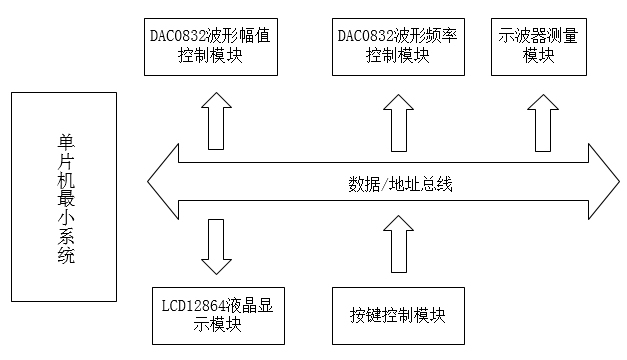仿真电路图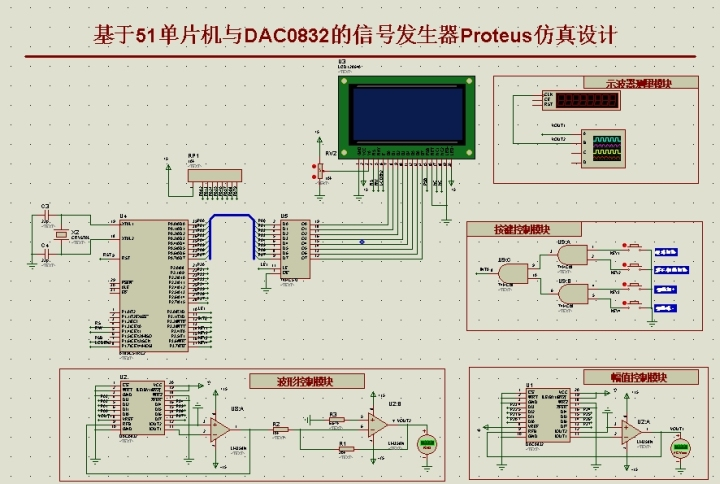仿真分析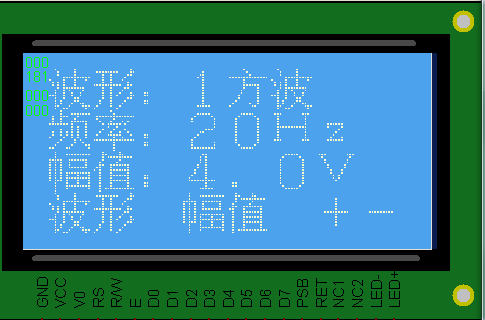图1 幅值为4V，频率为20Hz的方波信号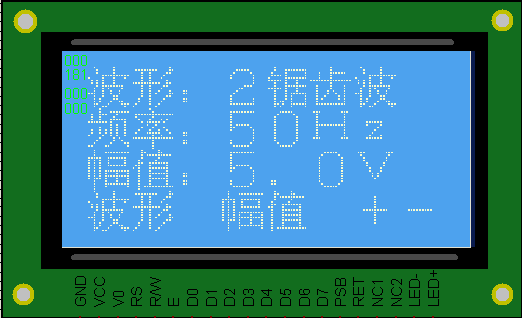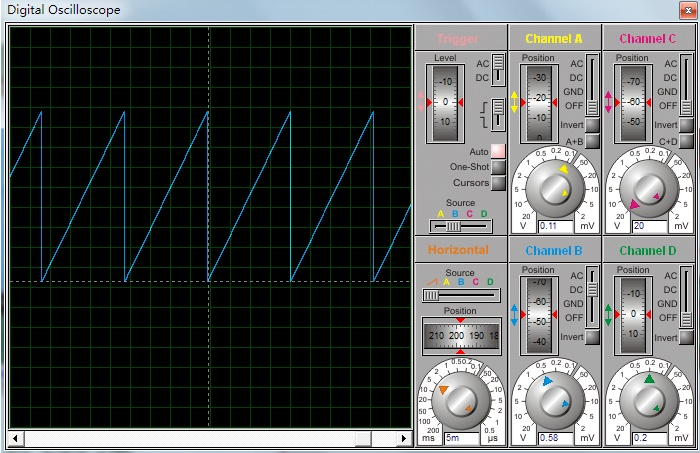图2 幅值为5V，频率为50Hz的锯齿波信号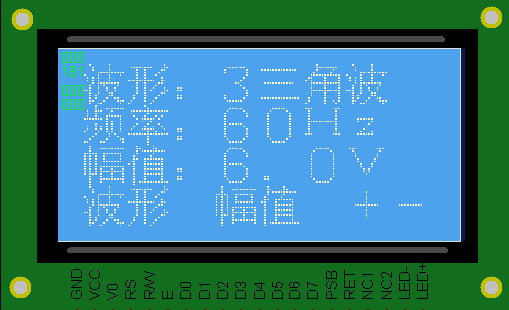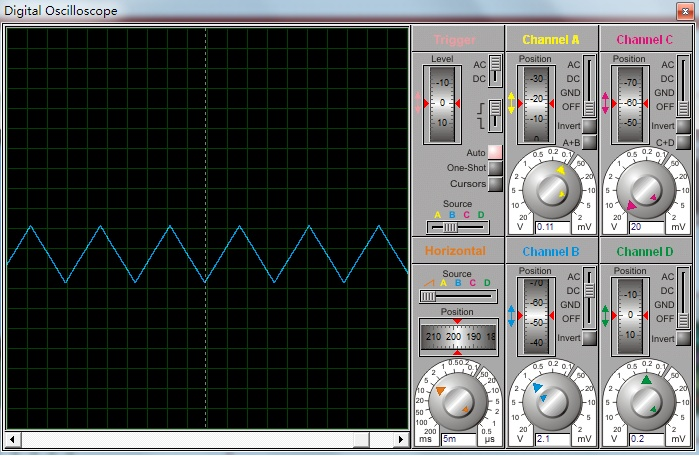图3 幅值为6V，频率为60Hz的三角波输出信号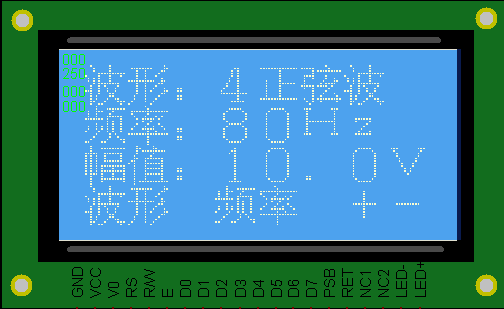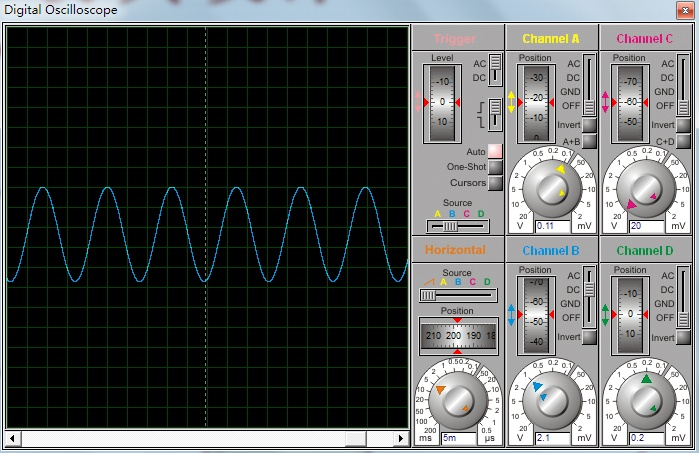图4 幅值为10V，频率为80Hz的正弦波输出信号

部分C程序

intmain

分享内容（1）基于51单片机与DAC0832的信号发生器设计论文完整版；（2）Proteus仿真文件（3）Keil C语言程序文件（4）DAC0832 技术手册（5）Visio绘制的流程图
资源截图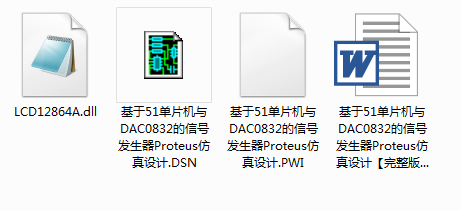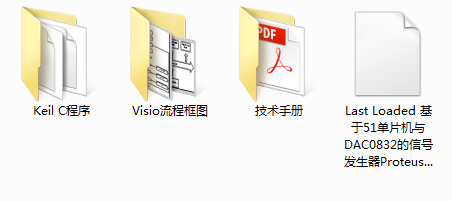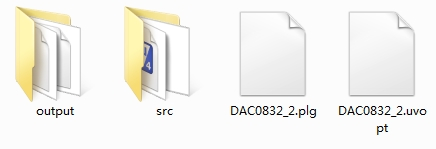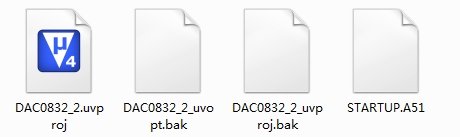由于本设计为作者原创设计，
获取完整的论文、Proteus仿真、Keil C程序、Visio流程图文件和技术手册等资料。
请搜索关注公众号（公众号请见头像处或个人简介，谢谢！）

展开全文• 本文借助easyx库函数（提供下载链接），很方便地用C语言实现了鼠标、按钮、进度条、坐标尺等操作，为普通C程序的友好人机交互界面提供了一种简单便捷的方案。


目录
0.引言1.素材准备2.编程2.1.创建你的界面2.2.创建按钮2.3.鼠标操作2.3.1.单击特效2.3.2.光标感应2.3.3.进度条

3.完整代码及效果

0.引言

看了CSDN上很多关于C程序图形化界面的介绍，有的代码繁琐难解，不方便调试修改；有的不够详细。本文提供的代码简单、易于移植、容易理解，望急需使用C语言制作图形化界面的朋友采纳。

对easyx尚不熟悉的朋友不需要担心，我敢打包票它只需10分钟就可以上手，而它为你节省的时间可能是3个小时甚至更多。关于easyx的简单应用请参考一篇我以前写的关于C程序可视化的博文。 →【C语言实现动画控制】←

本文的讲解是循序渐进的，读者应该重点关注每个步骤的理解，两步之间代码的变化，即可完全理解本文。
1.素材准备
easyx的下载链接如下：（本文使用的版本是2014冬至版） https://www.easyx.cn/downloads/ 注：使用easyx需要注意它兼容的编译器（下载的帮助文件会写），不同的easyx兼容的编译器不同，但总是和visual C++6兼容（和字符编码有关），本文以visual C++6编译器为例书写代码。easyx的最新英文帮助文档链接（下载2014冬至版会自带中文帮助文档）： https://docs.easyx.cn/en-us/intro如果你成功下载了easyx2014冬至版，那么解压后把头文件（easyx.h和graphic.h）和lib文件（amd64）分别放在VC文件夹默认的include文件夹和lib文件夹中。右键你的VC程序，选择打开文件所在位置，然后找到MFC文件夹，友情提供两个文件夹的位置截图。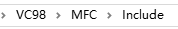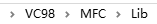建议编译的C文件以cpp后缀保存。
2.编程
2.1.创建你的界面

创建一个480×360的窗口，我们需要使用initgraph()函数，闲言少叙，让我们直接看一段代码：
#include <graphics.h>              // 引用图形库头文件
#include <conio.h>
#include <stdio.h>
#include <windows.h>				//用到了定时函数sleep()
#include <math.h>

int main()
{
int i;
short win_width,win_height;//定义窗口的宽度和高度
win_width = 480;win_height = 360;
initgraph(win_width,win_height);//初始化窗口（黑屏）
for(i=0;i<256;i+=5)
{
setbkcolor(RGB(i,i,i));//设置背景色，原来默认黑色
cleardevice();//清屏（取决于背景色）
Sleep(15);//延时15ms
}
closegraph();//关闭绘图界面
}



这段代码很容易理解，运行这段程序，就会出现逐渐明亮的屏幕。因为不断刷新背景色为

R

G

B

(

i

,

i

,

i

)

RGB(i,i,i)

。C语言中的颜色使用十六进制表示的，RGB函数可以将0~255范围内的三个整数三原色转换成这个十六进制。

cleardevice()函数用于清屏，是界面内所有元素都被清空，一般只会在初始化出现。

Sleep()是毫秒级延迟，当然界面变亮时间不一定是准确的15ms×255/5=0.765s，因为其他语句还需要执行时间。

closegraph()：关闭绘图界面。注意，如果初始化了绘图界面但没有在主函数结束前关闭它，可能会引发一些莫名其妙的错误！所以这个函数一定要有！
2.2.创建按钮

我们尝试在界面创建几个按钮，按钮需要的操作是绘制矩形和打印文字。虽然看着简单，但是里面还是有点学问，为了方便大家理解，还是先放上代码和注释。
#include <graphics.h>              // 引用图形库头文件
#include <conio.h>
#include <stdio.h>
#include <windows.h>				//用到了定时函数sleep()
#include <math.h>
int r1[]={30,20,130,60};//输入按钮的矩形参数
int r2[]={170,20,220,60};//运行按钮的矩形参数
int r3[]={260,20,310,60};//退出按钮的矩形参数
int main()
{
int i;
short win_width,win_height;//定义窗口的宽度和高度
win_width = 480;win_height = 360;
initgraph(win_width,win_height);//初始化窗口（黑屏）
for(i=0;i<256;i+=5)
{
setbkcolor(RGB(i,i,i));//设置背景色，原来默认黑色
cleardevice();//清屏（取决于背景色）
Sleep(15);//延时15ms
}
RECT R1={r1,r1,r1,r1};//矩形指针R1
RECT R2={r2,r2,r2,r2};//矩形指针R2
RECT R3={r3,r3,r3,r3};//矩形指针R3
LOGFONT f;//字体样式指针
gettextstyle(&f);					//获取字体样式
_tcscpy(f.lfFaceName,_T("宋体"));	//设置字体为宋体
f.lfQuality = ANTIALIASED_QUALITY;    // 设置输出效果为抗锯齿
settextstyle(&f);                     // 设置字体样式
settextcolor(BLACK);				//BLACK在graphic.h头文件里面被定义为黑色的颜色常量
drawtext("输入参数",&R1,DT_CENTER | DT_VCENTER | DT_SINGLELINE);//在矩形区域R1内输入文字，水平居中，垂直居中，单行显示
drawtext("运行",&R2,DT_CENTER | DT_VCENTER | DT_SINGLELINE);//在矩形区域R2内输入文字，水平居中，垂直居中，单行显示
drawtext("退出",&R3,DT_CENTER | DT_VCENTER | DT_SINGLELINE);//在矩形区域R3内输入文字，水平居中，垂直居中，单行显示
setlinecolor(BLACK);
rectangle(r1,r1,r1,r1);
rectangle(r2,r2,r2,r2);
rectangle(r3,r3,r3,r3);
system("pause");//暂停，为了显示
closegraph();
return 0;
}这里需要特别介绍的是矩形指针

p

R

e

c

t

pRect

，它使用句柄RECT定义，并且不可以中途再次赋值。之所以要设置矩形指针了为了打印字体的时候以矩形为边界自动填充。它的格式是RECT r={X1,Y1,X2,Y2}，X1和X2是矩形的左边和右边的横坐标，Y1和Y2是矩形的上边和下边的纵坐标，这一点和rectangle()绘制空心矩形函数参数排列一致。后面的DT_CENTER | DT_VCENTER | DT_SINGLELINE就是描述填充格式的常量。使用drawtext书写文字不需要再计算文字的坐标和设置大小，会方便很多。

LOGFONT是字体样式指针，通过gettextstyle()函数来获取当前的字体类型，再通过settextstyle()函数加以设置。这里只修改了字体的名称和显示质量，还可以修改斜体、下划线等属性，更详细的部分请参考帮助文档。
2.3.鼠标操作
2.3.1.单击特效

作为一个图形化界面的C程序，鼠标操作总不能少吧。在讲解程序前先别着急，简单为大家科普一下鼠标事件：

鼠标是输入设备，只要发生以下的事件，就会暂存在鼠标消息列表中，我们的操作系统就会依次响应列表中的鼠标消息事件，常用的鼠标事件如下：
WM_MOUSEMOVE——鼠标移动WM_MOUSEWHEEL——鼠标滚轮滚动WM_LBUTTONDOWN——鼠标左键按下WM_LBUTTONUP——鼠标左键弹起WM_LBUTTONDBLCLK——鼠标左键双击WM_RBUTTONDOWN——鼠标右键按下WM_RBUTTONUP——鼠标右键弹起WM_RBUTTONDBLCLK——鼠标左键双击WM_MBUTTONDOWN——鼠标中键按下WM_MBUTTONUP——鼠标中键弹起WM_MBUTTONDBLCLK——鼠标中键双击

我们只需要根据不断获取鼠标消息队列的消息并根据消息依次进行响应即可。

相信大家已经迫不及待了，那么请看下面一个简单的程序。
#include <graphics.h>              // 引用图形库头文件
#include <conio.h>
#include <stdio.h>
#include <windows.h>				//用到了定时函数sleep()
#include <math.h>
int r1[]={30,20,130,60};//输入按钮的矩形参数
int r2[]={170,20,220,60};//运行按钮的矩形参数
int r3[]={260,20,310,60};//退出按钮的矩形参数
int main()
{
int i;
short win_width,win_height;//定义窗口的宽度和高度
win_width = 480;win_height = 360;
initgraph(win_width,win_height);//初始化窗口（黑屏）
for(i=0;i<256;i+=5)
{
setbkcolor(RGB(i,i,i));//设置背景色，原来默认黑色
cleardevice();//清屏（取决于背景色）
Sleep(15);//延时15ms
}
RECT R1={r1,r1,r1,r1};//按钮1的矩形区域
RECT R2={r2,r2,r2,r2};//按钮2的矩形区域
RECT R3={r3,r3,r3,r3};//按钮2的矩形区域
LOGFONT f;
gettextstyle(&f);					//获取字体样式
_tcscpy(f.lfFaceName,_T("宋体"));	//设置字体为宋体
f.lfQuality = ANTIALIASED_QUALITY;    // 设置输出效果为抗锯齿
settextstyle(&f);                     // 设置字体样式
settextcolor(BLACK);				//BLACK在graphic.h头文件里面被定义为黑色的颜色常量
drawtext("输入参数",&R1,DT_CENTER | DT_VCENTER | DT_SINGLELINE);//在矩形区域R1内输入文字，水平居中，垂直居中，单行显示
drawtext("运行",&R2,DT_CENTER | DT_VCENTER | DT_SINGLELINE);//在矩形区域R2内输入文字，水平居中，垂直居中，单行显示
drawtext("退出",&R3,DT_CENTER | DT_VCENTER | DT_SINGLELINE);//在矩形区域R3内输入文字，水平居中，垂直居中，单行显示
setlinecolor(BLACK);
rectangle(r1,r1,r1,r1);
rectangle(r2,r2,r2,r2);
rectangle(r3,r3,r3,r3);
MOUSEMSG m;//鼠标指针
setrop2(R2_NOTXORPEN);//二元光栅——NOT(屏幕颜色 XOR 当前颜色)
while(true)
{
m = GetMouseMsg();//获取一条鼠标消息
if(m.uMsg==WM_LBUTTONDOWN)
{
for(i=0;i<=10;i++)
{
setlinecolor(RGB(25*i,25*i,25*i));//设置圆颜色
circle(m.x,m.y,2*i);
Sleep(25);//停顿2ms
circle(m.x,m.y,2*i);//抹去刚刚画的圆
}
FlushMouseMsgBuff();//清空鼠标消息缓存区
}
}
system("pause");//暂停，为了显示
closegraph();
return 0;
}每点击鼠标以下，应该可以看到鼠标点击处有一个逐渐扩大并淡出的圆（截图无法清晰，在画面的中右侧），当循环体内Sleep的视觉大于20ms后视觉效果很强。

每响应一次鼠标左键单击事件，都会调用一次清空鼠标消息缓存区的函数FlushMouseMsgBuff()，如果没有这个函数会怎么样呢？如果我们快速连续地单击鼠标左键N次，那么特效就会播放N次，如果特效播放速度比单击的速度慢，那么即使你停下来了，程序仍然会接着播放单击特效，因为你的左键单击仍然在鼠标的消息队列m.uMsg中的鼠标消息没有响应完。

这里需要解释的是一个二元光栅设置函数setrop2()，二元光栅是混合背景色和当前颜色的模式。我们这里采用的方式是同或（NOT XOR）的方式，若底色为白色（1），则当前颜色不变；若底色是黑色（0），则当前颜色反色。为什么需要采用这种方式呢？因为我们在第二次抹去原来的圆的时候不能采用白色，否则如果背景色原来就为黑（比如按钮和文字），就也会被抹成白色。而背景色与任意一个颜色同或两次都为其本身，即可起到还原背景色的效果。这里的背景色与cleardevice()前面那个背景色不同，这里的是指执行这一条绘画指令之前屏幕上的颜色。
2.3.2.光标感应

我们希望鼠标移到按钮上时按钮会有所变化，移开按钮时又会回到原样。这里我们采用一种简单的填充颜色的方法，就是按钮变色。我们需要解决一个问题就是按钮变色了但是按钮的文字不能被覆盖，那么我们还是需要使用到二元光栅。只是我们这次的模式改成了同或。

为了方便起见，存放三个按钮的数组我们合并为了一个二维数组，在鼠标事件中更容易使用和分配任务。
#include <graphics.h>              // 引用图形库头文件
#include <conio.h>
#include <stdio.h>
#include <windows.h>				//用到了定时函数sleep()
#include <math.h>
int r={{30,20,130,60},{170,20,220,60},{260,20,310,60}};//三个按钮的二维数组

int button_judge(int x,int y)
{
if(x>r && x<r && y>r && y<r)return 1;
if(x>r && x<r && y>r && y<r)return 2;
if(x>r && x<r && y>r && y<r)return 3;
return 0;
}
int main()
{
int i,event=0;
short win_width,win_height;//定义窗口的宽度和高度
win_width = 480;win_height = 360;
initgraph(win_width,win_height);//初始化窗口（黑屏）
for(i=0;i<256;i+=5)
{
setbkcolor(RGB(i,i,i));//设置背景色，原来默认黑色
cleardevice();//清屏（取决于背景色）
Sleep(15);//延时15ms
}
RECT R1={r,r,r,r};
RECT R2={r,r,r,r};
RECT R3={r,r,r,r};
LOGFONT f;
gettextstyle(&f);					//获取字体样式
_tcscpy(f.lfFaceName,_T("宋体"));	//设置字体为宋体
f.lfQuality = ANTIALIASED_QUALITY;    // 设置输出效果为抗锯齿
settextstyle(&f);                     // 设置字体样式
settextcolor(BLACK);				//BLACK在graphic.h头文件里面被定义为黑色的颜色常量
drawtext("输入参数",&R1,DT_CENTER | DT_VCENTER | DT_SINGLELINE);//在矩形区域R1内输入文字，水平居中，垂直居中，单行显示
drawtext("运行",&R2,DT_CENTER | DT_VCENTER | DT_SINGLELINE);//在矩形区域R2内输入文字，水平居中，垂直居中，单行显示
drawtext("退出",&R3,DT_CENTER | DT_VCENTER | DT_SINGLELINE);//在矩形区域R3内输入文字，水平居中，垂直居中，单行显示
setlinecolor(BLACK);
rectangle(r,r,r,r);
rectangle(r,r,r,r);
rectangle(r,r,r,r);
MOUSEMSG m;//鼠标指针

while(true)
{
m = GetMouseMsg();//获取一条鼠标消息

switch(m.uMsg)
{
case WM_MOUSEMOVE:
setrop2(R2_XORPEN);
setlinecolor(LIGHTCYAN);//线条颜色为亮青色
setlinestyle(PS_SOLID, 3);//设置画线样式为实现，10磅
setfillcolor(WHITE);//填充颜色为白色
if(button_judge(m.x,m.y)!=0)
{
if(event != button_judge(m.x,m.y))
{
event = button_judge(m.x,m.y);//记录这一次触发的按钮
fillrectangle(r[event-1],r[event-1],r[event-1],r[event-1]);//有框填充矩形（X1,Y1,X2,Y2）
}
}
else
{
if(event != 0)//上次触发的按钮未被修正为原来的颜色
{
fillrectangle(r[event-1],r[event-1],r[event-1],r[event-1]);//两次同或为原来颜色
event = 0;
}
}
break;
case WM_LBUTTONDOWN:
setrop2(R2_NOTXORPEN);//二元光栅——NOT(屏幕颜色 XOR 当前颜色)
for(i=0;i<=10;i++)
{
setlinecolor(RGB(25*i,25*i,25*i));//设置圆颜色
circle(m.x,m.y,2*i);
Sleep(30);//停顿30ms
circle(m.x,m.y,2*i);//抹去刚刚画的圆
}
break;
FlushMouseMsgBuff();//清空鼠标消息缓存区
}
}
system("pause");//暂停，为了显示
return 0;
}这里我们运用了两次设置二元光栅的函数setrop2，在鼠标的移动条件内(case MOUSEMOVE)，我们使用的是屏幕颜色和当前颜色异或，这是为什么呢？因为fillrectangle()函数是画一个有框填充矩形，我们让这个有框填充矩形和原按钮的一样大。由于线条的颜色为亮青色，填充颜色为白色（1），白色的填充颜色和屏幕颜色异或，取的是屏幕颜色的反色。按钮的边框是黑色（0），它与亮青色异或，则会保留原来的亮青色。

与同或一样，异或两次等于没有执行操作，所以可以还原到原屏幕画布的颜色。
2.3.3.进度条

既然涉及到进度条了，那么就应该涉及到正式程序了，这里我不想涉及太多的专业知识影响大家理解，但又不能让程序运行得太快以至于看不出进度条的变化，所以我设计了一个简单的弹性球轨迹作图程序。

假设球半径为R，初始高度为

h

0

h_0

，初速度为0（自由落体），非弹性碰撞时能量损失率为

α

\alpha

。计算部分子函数如下：
int simulation()
{
float dt = 0.01;//仿真间隔10ms
long int N = (long int)(sim_t/dt);//迭代次数
float *h=(float*)calloc(N,sizeof(float));//高度
float *v=(float*)calloc(N,sizeof(float));//速度（竖直方向）
long int i;//迭代变量
for(i=1;i<N;i++)
{
if(h[i-1]>R)//未发生碰撞
{
v[i]=v[i-1]-9.8*dt;//速度计算
}
else//发生碰撞，动能损失alpha，速度损失alpha的开方
{
v[i]=-sqrt(alpha)*v[i-1];
}
}
free(h);
free(v);//释放内存
return 0;
}


当然，我们还需要绘图网格，定义绘图网格的函数如下：
void init_figure()
{
int i;
setrop2(R2_COPYPEN);//当前颜色
setlinecolor(BLACK);
setlinestyle(PS_SOLID);//实线
rectangle(30,100,420,330);//外框线
setlinestyle(PS_DOT);//点线
for(i=30+39;i<420;i+=39)
{
line(i,100,i,330);//竖直辅助线
}
for(i=100+23;i<330;i+=23)
{
line(30,i,420,i);//水平辅助线
}
}


注意，我们使用了rectangle()空心矩形函数绘制网格外框架，使用了line函数依次画出了辅助线。

我们现在的目标就是将h的坐标转换到网格上去，绘制出球心的轨迹，这样似乎并不复杂，只需要对simulation()函数稍加修改即可。
int simulation()
{
float dt = 0.01;//仿真间隔10ms
float dy = 230/h0;//单位纵坐标
long int N = (long int)(sim_t/dt);//迭代次数
float *h=(float*)calloc(N,sizeof(float));//高度
float *v=(float*)calloc(N,sizeof(float));//速度（竖直方向）
long int i;//迭代变量
float process_duty;//进度
init_figure();//初始化图像网格
setrop2(R2_COPYPEN);//当前颜色
//计算步骤
h=h0;v=0;
for(i=1;i<N;i++)
{
if(h[i-1]>R)//未发生碰撞
{
v[i]=v[i-1]-9.8*dt;//速度计算
}
else//发生碰撞，动能损失alpha，速度损失alpha的开方
{
v[i]=-sqrt(alpha)*v[i-1];
}
h[i]=h[i-1]+v[i]*dt;//高度计算
process_duty = (i+1)/(float)(N);
putpixel(30+(int)(process_duty*390),330-(int)(h[i]*dy),RED);//画点putpixel(X,Y,color*)
Sleep(dt*1000);//延时
}
free(h);
free(v);
return 0;
}


这里的新函数putpixel（X,Y,color*）是画像素点的函数，适合刻画不连续或不规则的移动轨迹。

现在我们只剩下了刻画进度条的函数了，进度条的刷新很明显是应该放在for循环里面的，那么我们采用什么进度条的格式呢？进度条可以有圆形、扇形、长条连续型、长条不连续型等多种，我们这里采用的是环形进度条，将进度数字显示在环中心。请看以下的对simulation()函数改进的代码：
//仿真运行
int simulation()
{
char t;//百分值的字符
char *out_text;//带百分号的百分字符
float dt = 0.01;//仿真间隔10ms
float dy = 230/h0;//单位纵坐标
long int N = (long int)(sim_t/dt);//迭代次数
float *h=(float*)calloc(N,sizeof(float));//高度
float *v=(float*)calloc(N,sizeof(float));//速度（竖直方向）
long int i;//迭代变量
float process_duty;//进度
RECT r={370,35,400,65};//百分值显示区域的矩形指针
init_figure();//初始化图像网格
setrop2(R2_COPYPEN);//当前颜色
setfillcolor(WHITE);
setlinecolor(WHITE);
fillrectangle(354,19,411,81);//覆盖原进度条区域
setlinestyle(PS_NULL);//无线条
setbkmode(TRANSPARENT);//设置文字填充背景为透明
//计算步骤
h=h0;v=0;
BeginBatchDraw();//开始缓存区
for(i=1;i<N;i++)
{
if(h[i-1]>R)//未发生碰撞
{
v[i]=v[i-1]-9.8*dt;//速度计算
}
else//发生碰撞，动能损失alpha，速度损失alpha的开方
{
v[i]=-sqrt(alpha)*v[i-1];
}
setfillcolor(WHITE);
setlinecolor(WHITE);
fillrectangle(354,19,416,81);//覆盖原进度条区域
h[i]=h[i-1]+v[i]*dt;//高度计算
process_duty = (i+1)/(float)(N);
setlinestyle(PS_SOLID);
putpixel(30+(int)(process_duty*390),330-(int)(h[i]*dy),RED);
setfillcolor(BLUE);
setlinestyle(PS_NULL);
fillpie(355,20,415,80,0,process_duty*2*PI);
setfillcolor(WHITE);
fillcircle(385,50,20);
sprintf(t,"%d",(int)(process_duty*100.0));//整型转换为字符串
out_text = strcat(t,"%");//添加一个百分号
drawtext(out_text,&r,DT_CENTER | DT_VCENTER | DT_SINGLELINE);
Sleep(dt*1000);
FlushBatchDraw();//刷新缓存区
}
EndBatchDraw();//结束缓存区
free(h);
free(v);
return 0;
}


这里我们需要多加载一个头文件<string.h>。

首先需要计算进度条的坐标，把环形进度条区域用白色矩形刷新掉，环形进度条需要一个扇形和圆形的组合，扇形的角度是0~360°。这里我们用到了fillpie(X1,Y1,X2,Y2,start_angle,end_angle)，前四个参数为椭圆扇形的外接矩形坐标，后两个参数分别为起始角和终止角（弧度制）。每过一次迭代都重新计算终止角（起始角始终为0），即可起到扇形角度逐渐增长的效果，再用一个白色填充圆覆盖中心部分即可变成环形进度条。

FlushBatchDraw()函数是刷新缓存区的函数，与BeginBatchDraw()和EndBatchDraw()一起使用，如果我们绘图之后不想立即显示，而想批量绘图最后一起刷新画板，用缓存区的方法再合适不过了。
3.完整代码及效果
#include <graphics.h>              // 引用图形库头文件
#include <conio.h>
#include <stdio.h>
#include <windows.h>				//用到了定时函数sleep()
#include <math.h>
#include <string.h>
#define PI 3.1416
int r={{30,20,130,60},{170,20,220,60},{260,20,310,60}};//三个按钮的二维数组
float alpha,R,h0,sim_t;//碰撞时的能量损失率，球的半径、初始高度、仿真时间
//按钮判断函数
int button_judge(int x,int y)
{
if(x>r && x<r && y>r && y<r)return 1;
if(x>r && x<r && y>r && y<r)return 2;
if(x>r && x<r && y>r && y<r)return 3;
return 0;
}
//初始化图像
void init_figure()
{
int i;
setrop2(R2_COPYPEN);//当前颜色
setlinecolor(BLACK);
setlinestyle(PS_SOLID);//实线
rectangle(30,100,420,330);//外框线
setlinestyle(PS_DOT);//点线
for(i=30+39;i<420;i+=39)
{
line(i,100,i,330);//竖直辅助线
}
for(i=100+23;i<330;i+=23)
{
line(30,i,420,i);//水平辅助线
}
}
//仿真运行
int simulation()
{
char t;//百分值的字符
char *out_text;
float dt = 0.01;//仿真间隔10ms
float dy = 230/h0;//单位纵坐标
long int N = (long int)(sim_t/dt);//迭代次数
float *h=(float*)calloc(N,sizeof(float));//高度
float *v=(float*)calloc(N,sizeof(float));//速度（竖直方向）
long int i;//迭代变量
float process_duty;//进度
RECT r={370,35,400,65};//百分值显示区域的矩形指针
init_figure();//初始化图像网格
setrop2(R2_COPYPEN);//当前颜色
setfillcolor(WHITE);
setlinecolor(WHITE);
fillrectangle(354,19,411,81);//覆盖原进度条区域
setlinestyle(PS_NULL);//无线条
setbkmode(TRANSPARENT);//设置文字填充背景为透明
//计算步骤
h=h0;v=0;
BeginBatchDraw();//开始缓存区
for(i=1;i<N;i++)
{
if(h[i-1]>R)//未发生碰撞
{
v[i]=v[i-1]-9.8*dt;//速度计算
}
else//发生碰撞，动能损失alpha，速度损失alpha的开方
{
v[i]=-sqrt(alpha)*v[i-1];
}
setfillcolor(WHITE);
setlinecolor(WHITE);
fillrectangle(354,19,416,81);//覆盖原进度条区域
h[i]=h[i-1]+v[i]*dt;//高度计算
process_duty = (i+1)/(float)(N);
setlinestyle(PS_SOLID);
putpixel(30+(int)(process_duty*390),330-(int)(h[i]*dy),RED);
setfillcolor(BLUE);
setlinestyle(PS_NULL);
fillpie(355,20,415,80,0,process_duty*2*PI);
setfillcolor(WHITE);
fillcircle(385,50,20);
sprintf(t,"%d",(int)(process_duty*100.0));//整型转换为字符串
out_text = strcat(t,"%");//添加一个百分号
drawtext(out_text,&r,DT_CENTER | DT_VCENTER | DT_SINGLELINE);
Sleep(dt*1000);
FlushBatchDraw();//刷新缓存区
}
EndBatchDraw();//结束缓存区
free(h);
free(v);
return 0;
}

int main()
{
int i,event=0;
char s;//输入字符串变量
short win_width,win_height;//定义窗口的宽度和高度
win_width = 480;win_height = 360;
initgraph(win_width,win_height);//初始化窗口（黑屏）
for(i=0;i<256;i+=5)
{
setbkcolor(RGB(i,i,i));//设置背景色，原来默认黑色
cleardevice();//清屏（取决于背景色）
Sleep(30);//延时30ms
}
RECT R1={r,r,r,r};
RECT R2={r,r,r,r};
RECT R3={r,r,r,r};
LOGFONT f;//字体样式指针
gettextstyle(&f);					//获取字体样式
_tcscpy(f.lfFaceName,_T("宋体"));	//设置字体为宋体
f.lfQuality = ANTIALIASED_QUALITY;    // 设置输出效果为抗锯齿
settextstyle(&f);                     // 设置字体样式
settextcolor(BLACK);				//BLACK在graphic.h头文件里面被定义为黑色的颜色常量
drawtext("输入参数",&R1,DT_CENTER | DT_VCENTER | DT_SINGLELINE);//在矩形区域R1内输入文字，水平居中，垂直居中，单行显示
drawtext("运行",&R2,DT_CENTER | DT_VCENTER | DT_SINGLELINE);//在矩形区域R2内输入文字，水平居中，垂直居中，单行显示
drawtext("退出",&R3,DT_CENTER | DT_VCENTER | DT_SINGLELINE);//在矩形区域R3内输入文字，水平居中，垂直居中，单行显示
setlinecolor(BLACK);
rectangle(r,r,r,r);
rectangle(r,r,r,r);
rectangle(r,r,r,r);
MOUSEMSG m;//鼠标指针

while(true)
{
m = GetMouseMsg();//获取一条鼠标消息

switch(m.uMsg)
{
case WM_MOUSEMOVE:
setrop2(R2_XORPEN);
setlinecolor(LIGHTCYAN);//线条颜色为亮青色
setlinestyle(PS_SOLID, 3);//设置画线样式为实现，10磅
setfillcolor(WHITE);//填充颜色为白色
if(button_judge(m.x,m.y)!=0)
{
if(event != button_judge(m.x,m.y))
{
event = button_judge(m.x,m.y);//记录这一次触发的按钮
fillrectangle(r[event-1],r[event-1],r[event-1],r[event-1]);//有框填充矩形（X1,Y1,X2,Y2）
}
}
else
{
if(event!=0)//上次触发的按钮未被修正为原来的颜色
{
fillrectangle(r[event-1],r[event-1],r[event-1],r[event-1]);//两次同或为原来颜色
event = 0;
}
}
break;
case WM_LBUTTONDOWN:
setrop2(R2_NOTXORPEN);//二元光栅——NOT(屏幕颜色 XOR 当前颜色)
for(i=0;i<=10;i++)
{
setlinecolor(RGB(25*i,25*i,25*i));//设置圆颜色
circle(m.x,m.y,2*i);
Sleep(20);//停顿30ms
circle(m.x,m.y,2*i);//抹去刚刚画的圆
}
//按照按钮判断左键单击后的操作
switch(button_judge(m.x,m.y))
{
//复原按钮原型
case 1:
InputBox(s,30,"请输入碰撞时的能量损失率、球的半径、初始高度、仿真时间");
sscanf(s,"%f%f%f%f",&alpha,&R,&h0,&sim_t);//将输入字符串依次扫描到全局变量里面
FlushMouseMsgBuffer();//单击事件后清空鼠标消息
break;
case 2:
simulation();//仿真运行
FlushMouseMsgBuffer();//单击事件后清空鼠标消息
break;
case 3:
closegraph();//关闭绘图环境
exit(0);//正常退出
default:
FlushMouseMsgBuffer();//单击事件后清空鼠标消息
//printf("\r\n(%d,%d)",m.x,m.y);//打印鼠标坐标，方便调试时确定区域
break;
}
break;
}
}
return 0;
}希望本文对您有帮助，谢谢阅读。
展开全文图形化界面 控制台 可视化
• C语言结合gnuplot绘制锯齿


文章目录
C程序gnuplot绘图matlab代码matlab绘图

C程序
#include<stdio.h>
#define pi 3.1415926
main()
{
int i;
double s, t;
for(i = 0; i <= 800*pi; i++)	//因为t=i/100.0，所以t的取值范围为[0,8*pi]
{
t = i / 100.0;	//pi个单位内取100个样本点
if(t>=0 && t<=2*pi)
s = 1/pi*t-1;
if(t>2*pi && t<=4*pi)
s = 1/pi*t-3;
if(t>4*pi && t<=6*pi)
s = 1/pi*t-5;
if(t>6*pi && t<=8*pi)
s = 1/pi*t-7;
printf("%f\t%f\n", t, s);
}
}
gnuplot绘图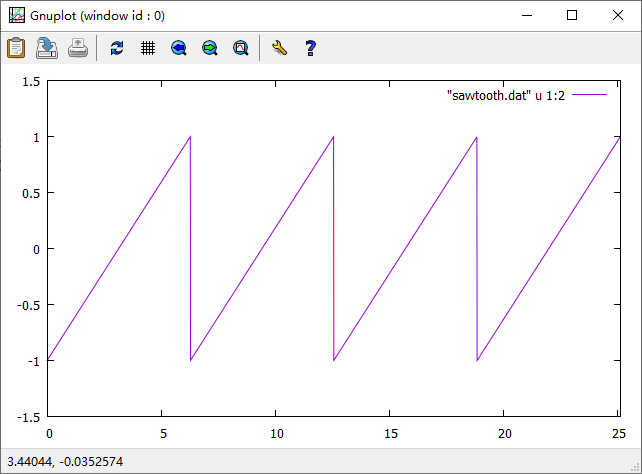matlab代码
x = 0 : 0.01 :8*pi;
y = sawtooth(x);
plot(x, y);
axis([0 8*pi -1.5 1.5])

matlab绘图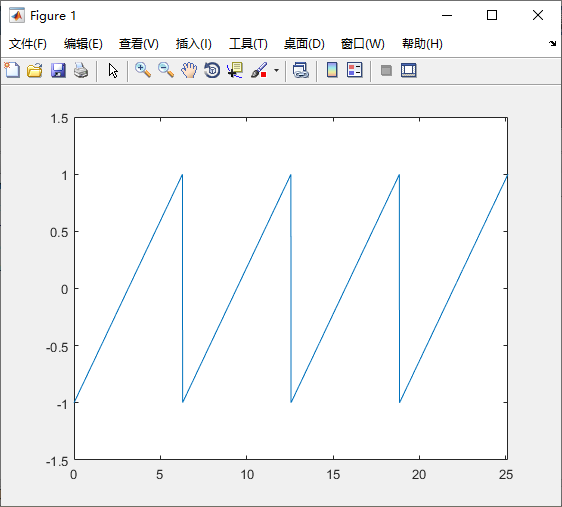展开全文• 输出最终的锯齿数组。初始时矩阵为空。输入格式第一行输入两个整数 n,m(1≤n,m≤10000)n,m(1 \leq n,m \leq 10000)n,m(1≤n,m≤10000)，其中 nnn 表示锯齿数组的行数，mmm 表示插入的元素总数。接下来一共 ...
• 如果符合下列情况之一，则数组 A 就是 锯齿数组： 每个偶数索引对应的元素都大于相邻的元素，即 A > A < A > A < A > ... 或者，每个奇数索引对应的元素都大于相邻的元素，即 A[0...
• 给定一个二叉树，返回其节点值的锯齿形层次遍历。（即先从左往右，再从右往左进行下一层遍历，以此类推，层与层之间交替进行）。 例如： 给定二叉树 [3,9,20,null,null,15,7], 3 / 9 20 / 15 7 返回锯齿形层次遍历...
• 当画一些不规则图形时，经常会出现锯齿，本人就曾遇到这样的问题，不知该如何下手，想尽种种办法后，终于解决了。
• 使用单片机实现PCF8591输出锯齿波的C语言实例免费下载
• 如果符合下列情况之一，则数组A就是 锯齿数组： 每个偶数索引对应的元素都大于相邻的元素，即A > A < A > A < A > ...或者，每个奇数索引对应的元素都大于相邻的元素，即A < A...
• 1.输出4种波形：方波、锯齿波、三角波和正弦波； 2.幅值调节范围为：2V-10V; 3.频率调节范围为：20Hz-80Hz; 内容简介 信号发生器仿真系统由51单片机最小系统、LCD12864液晶模块、DAC0832波形转换模块（包括波形幅值...DAC0832 信号发生器 波形发生器 51单片机 Proteus仿真
• 要求：给定一个二叉树，返回其节点值的锯齿形层次遍历。（即先从左往右，再从右往左进行下一层遍历，以此类推，层与层之间交替进行）。 （这个题目就是在LeetCode-102基础上添加了要求，基本思路大致相同） 示例 ...LeetCode
• 关于锯齿矩阵，咱们先来看看题目要求： 最近在参与计蒜客的实践，本题是关于链表，但小编想了好久（技术不精），还是没做出来，于是换成了自己比较习惯的结构体。 原理： 首先，我们需要记录需要插入的整数对应的...
• 单片机的锯齿波波形的设计与应用，设计方式等等等等
• 输入函数 1.cin 1）cin >> a、cin.get、cin.getline均返回一个cin对象，故可以连写cin.get.getline...； cin在适当的位置可以转换为bool类型，例如while(cin >...2) cin遇分隔符结束，并且自动忽略分隔符...
• Problem Description Give you a string, just circumgyrate. The number N means you just circumgyrate the string N times, and each time you circumgyrate the string for 45 degree anticlockwise. ...Golang erlang r语言
• 利用c51Proteus仿真输出反锯齿波的c语言程序
• /* * 算法思想： * 与102题目一样，102的结果，第i&1 != 0 行，反转即可。 * * */ #define LEV 1024 ...void get(Node *node, int level, int **ret, int *ret_index, int *rcs){ ... ...
• 二叉树的锯齿形层次遍历 这个程序没写出来，摘抄了九章算法的代码，方便复习整理。 https://www.jiuzhang.com/solutions/binary-tree-zigzag-level-order-traversal/ 核心思想 利用stack的办法解决。也可以用普通...二叉树 算法
• 单片机系统中文字显示部分一般用的是用取模软件取出字模后输出到屏幕上显示，这种方式显示的字体有锯齿，在DPI低的显示屏上锯齿效果非常明显。对于单色屏来说，这种情况无解。但是对于彩屏来说，如果还是用这种方式...单片机 平滑 字体 alphablend
• C语言实现ARC圆弧插补计算,有用得到的朋友可以试试圆弧插补
• C语言程序格式特点 -习惯用小写字母，大小写敏感 -不使用行号，无程序行概念 -可使用空行和空格 -输入输出由库函数实现 -常用锯齿形书写格式 main() { int i,j,sum; sum=0; for(i=1;i;i++) { for(j=1;j;j++) ...c# c++
• 1.用C语言产生均匀分布的随机数 (1)功能 产生（a，b）区间上均匀分布的随机数 (2)函数简介 均匀分布函数的概率密度函数为 f(x)={1b−a，a≤x≤b0，其它 f(x)=\begin{cases} \frac{1}{b-a} ，a\leq x\leq b\\ 0，其它...
• 用的是直通方式，程序非常简单一看就懂，通过对程序的修改可产生方波，三角波等...

# c语言锯齿c语言 订阅